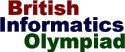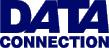The British Informatics Olympiad is the computing competition for schools and colleges. We are proud to present BIO'99.The 1999 British Informatics Olympiad Final
Zig-zag numbers

A zig-zag number is one where the digits alternatively rise and fall, always rising initially. For example, there are sixteen zig-zag numbers using the digits 1,...,5:

```13254   15342   25143   35142
14253   23154   25341   35241
14352   24153   34152   45132
15243   24351   34251   45231
```

Write a program that inputs two numbers : b (1<=b<=15) then n. You should calculate the nth zig-zag number (in increasing numerical order) that uses the digits 1,...,b once each. If there are Zb such zig-zag numbers, you will always be given 1<=n<=Zb. Z15 fits into a signed 32-bit integer.

`5 10`

`25341`

### Sample Input 2

`15 50000000`

### Sample Output 2

`185ebc9fad46273`